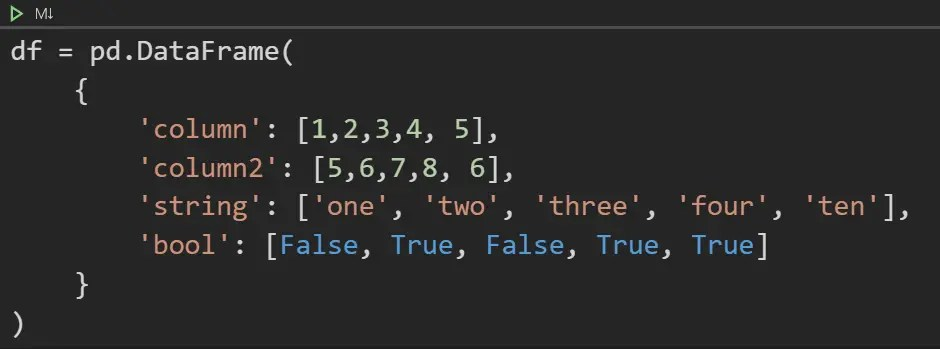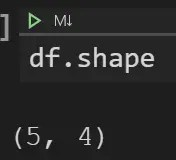# How to Create a New DataFrame in Python using Pandas

This tutorial will teach you how to create new columns and datasets in python using pandas for data analysis. There are multiple tools that you can use to create a new dataframe, but pandas is one of the easiest and most popular tools to create datasets. Let’s dive in.

### Step 1: Import pandas### Step 2: Use the pandas dataframe function to define your columns and the values that is stored in each column.WARNING!!! Make sure that all the columns have the same number of datapoints.

For example, if “column” was = [1,2,3,4,],

then executing the code will throw an error because “column” is one datapoint shorter than all the other columns.

All the datapoints have to be the same length which means same number of rows for all columns.

### Step 3: Verify that the dataframe creation was successful.

This is how you preview the first 5 rows of a dataset using pandas and python.

The dataframe is automatically assigned an index starting from 0. And the data we defined above has been put into a table format by the pandas dataframe function.

### Step 4: Check the shape of the dataset to make sure that is what you expect.This is how you check the shape of a dataset or csv file using python and pandas.

In this case, we created 4 columns with 5 data rows each. The shape function verifies this information for us.

When using “dataframe.shape”: 1st number = number of rows, 2nd number = number of columns

### Step 5: Check the data types of the dataframe to verify it is what is expected.This is how you check the type of data you have in a dataset using python and pandas.

Numbers are called “Int” which is Integers

Texts/strings are called “Object

True/False are called “bool” which is boolean

Scroll to Top